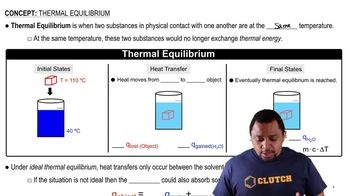Start typing, then use the up and down arrows to select an option from the list.
8:07 minutes
Problem 69
Textbook Question

# Two substances, A and B, initially at different temperatures, come into contact and reach thermal equilibrium. The mass of substance A is 6.15 g and its initial temperature is 20.5 °C. The mass of substance B is 25.2 g and its initial temperature is 52.7 °C. The final temperature of both substances at thermal equilibrium is 46.7 °C. If the specific heat capacity of substance B is 1.17 J>g # °C, what is the specific heat capacity of substance A?Verified Solution
This video solution was recommended by our tutors as helpful for the problem above.
427views